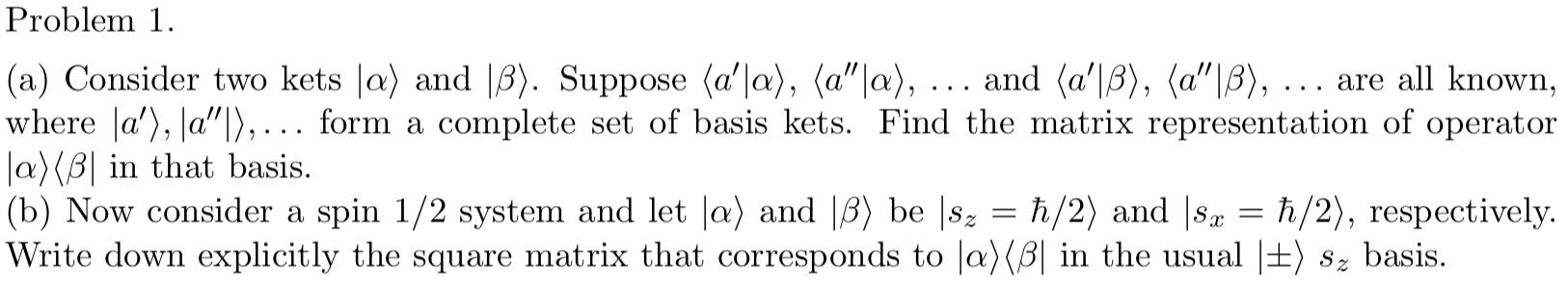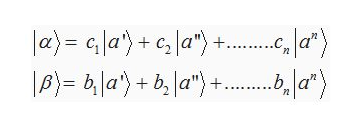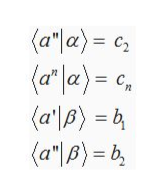Problem 1|(a) Consider two kets |a) and |3). Suppose (a'|a), (a"|a), .. . and (a'|B), (a"|B), ...where a'), a"|),... form aJa)(B| in that basis|(b) Now consider a spin 1/2 system and let |a) and |B) be |8|Write down explicitly the square matrix that corresponds to |a)(B| in the usual |±) 8z basis.are all known,complete set of basis kets. Find the matrix representation of operatorh/2) and |sah/2), respectively.

Questionhelp_outlineImage TranscriptioncloseProblem 1 |(a) Consider two kets |a) and |3). Suppose (a'|a), (a"|a), .. . and (a'|B), (a"|B), ... where a'), a"|),... form a Ja)(B| in that basis |(b) Now consider a spin 1/2 system and let |a) and |B) be |8 |Write down explicitly the square matrix that corresponds to |a)(B| in the usual |±) 8z basis. are all known, complete set of basis kets. Find the matrix representation of operator h/2) and |sa h/2), respectively. fullscreen
Step 1

a)

The kets  |α> and  |β> can be expanded  in terms on their basis vectors as,help_outlineImage Transcriptionclosea)= la'+c,la") +.. p)= ba')b a") 2 fullscreen
Step 2

Here c1,c2…cn  and b1.b2….bn  are the amplitudes of the projection of  the ket  |α> along their respective basis vectors .

The above representation can be further written in a more compact form by finding the amplitudes of projection mentioned above.

c1 is computed  by operation of the bra <a’| to the expression for |α> on both sides as follows

Step 3

Similarly c2…cn  and b1.b2….bn  can also be...help_outlineImage Transcriptionclose(a"|a)= c = by (a'B) a"|B)= b fullscreen

Want to see the full answer?

See Solution

Want to see this answer and more?

Our solutions are written by experts, many with advanced degrees, and available 24/7

See Solution
Tagged in

Science